Next: Volume Integrals Up: Vectors Previous: Line Integrals

## Surface Integrals

Surface integrals often arise in Physics. For instance, the rate of flow of a liquid of velocity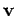through an infinitesimal surface of vector area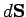is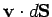(i.e., the product of the normal component of the velocity,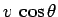, and the magnitude of the area,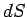, whereis the angle subtended betweenand). The net rate of flow through a surfacemade up of very many infinitesimal surfaces is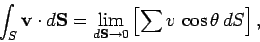(48)

whereis the angle subtended between a surface elementand the local flow velocity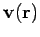. If the surface is closed, and the surface elements all point outward, then the integral is conventionally written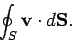(49)

In this case, the integral is often termed the flux of the velocity fieldout of the closed surface.

Richard Fitzpatrick 2007-07-14